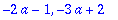Solving Quadratic Equations with Maple

First we use the well known solution formula of the equation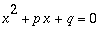> x1 := -p/2 + sqrt((p/2)^2 -q);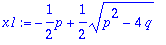> x2 := -p/2 - sqrt((p/2)^2 -q);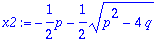Now we test it with numerical values:

> p:=1: q:=-6:

> x1;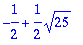> simplify(%);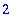> simplify(x2);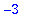Next we use the formula with algebraic expressions:

> p := 5*a-1: q := 6*a^2-a-2:

> x1;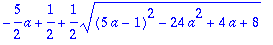> simplify(%);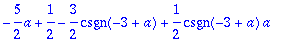To further simplify this expression, we need additional information about a, e.g.

> assume(a>=3);

> simplify(x1);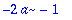> simplify(x2);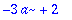What happens in the other case?

> assume(a<3);

> simplify(x1);> simplify(x2);Of course Maple can solve quadratic equations itself:

> restart;

> QE := x^2+p*x+q:

> solve(QE, x);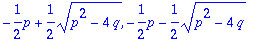> p :=1:q:=-6:

> solve(QE, x);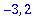> p := 5*a-1: q := 6*a^2-a-2:

> solve(QE, x);